# 1451 Star of Wonder

An important part of Mathematics is noticing patterns. I love it when mathematicians ask students, “What do you notice? What do you wonder?”

Those are questions you can ponder as you gaze on this star of wonder made from several different graphs.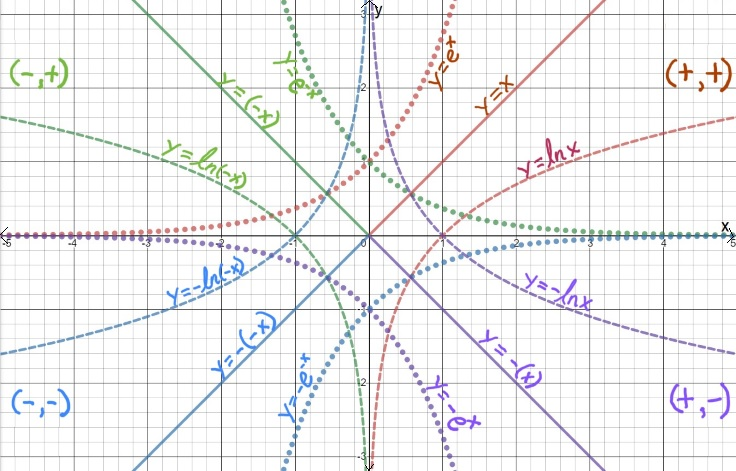To help distinguish the graphs, the dotted lines are exponential functions, the dashed lines are natural logarithm functions, and the solid lines are linear functions.

What do you notice? What do you wonder?

Now I’ll tell you a little bit about the post number, 1451:

• 1451 is a prime number.
• Prime factorization: 1451 is prime.
• 1451 has no exponents greater than 1 in its prime factorization, so √1451 cannot be simplified.
• The exponent in the prime factorization is 1. Adding one to that exponent we get (1 + 1) = 2. Therefore 1451 has exactly 2 factors.
• The factors of 1451 are outlined with their factor pair partners in the graphic below.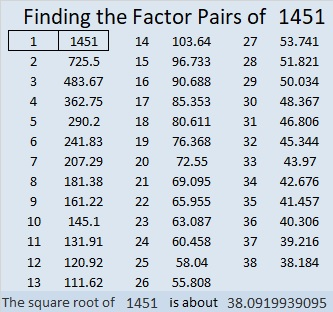How do we know that 1451 is a prime number? If 1451 were not a prime number, then it would be divisible by at least one prime number less than or equal to √1451. Since 1451 cannot be divided evenly by 2, 3, 5, 7, 11, 13, 17, 19, 23, 29 31, or 37, we know that 1451 is a prime number.

# 1439 and Level 6

You know the factors of 49, but to complete this puzzle, you will also have to determine the answers to a few questions:

Will the common factor of 24 and 32 be 4 or 8?
Will the common factor of 20 and 40 be 4, 5, or 10?
Will the common factor of 27 and 9 be 3 or 9?

Don’t guess and check! Look at the other clues, and some of the possibilities will be eliminated*. Once you’ve found the next clue to use, continue using logic until the whole puzzle is completed.Print the puzzles or type the solution in this excel file: 10 Factors 1432-1442

• 1439 is a prime number.
• Prime factorization: 1439 is prime.
• 1439 has no exponents greater than 1 in its prime factorization, so √1439 cannot be simplified.
• The exponent in the prime factorization is 1. Adding one to that exponent we get (1 + 1) = 2. Therefore 1439 has exactly 2 factors.
• The factors of 1439 are outlined with their factor pair partners in the graphic below.How do we know that 1439 is a prime number? If 1439 were not a prime number, then it would be divisible by at least one prime number less than or equal to √1439. Since 1439 cannot be divided evenly by 2, 3, 5, 7, 11, 13, 17, 19, 23, 29 31, or 37, we know that 1439 is a prime number.

* Did you notice that either one of the 24’s or 6 must use a 3 because only two 6s are allowed to be used as factors? What does that tell you about the common factor of 27 and 9?

# 1429 Find the Factors Challenge

I wanted today’s puzzle to look like a big candy bar, but I don’t think I succeeded. I hope you will still think it is the best treat you got today! Good luck!Print the puzzles or type the solution in this excel file: 12 Factors 1419-1429

Now I’ll tell you some facts about the number 1429:

• 1429 is a prime number.
• Prime factorization: 1429 is prime.
• 1429 has no exponents greater than 1 in its prime factorization, so √1429 cannot be simplified.
• The exponent in the prime factorization is 1. Adding one to that exponent we get (1 + 1) = 2. Therefore 1429 has exactly 2 factors.
• The factors of 1429 are outlined with their factor pair partners in the graphic below.

How do we know that 1429 is a prime number? If 1429 were not a prime number, then it would be divisible by at least one prime number less than or equal to √1429. Since 1429 cannot be divided evenly by 2, 3, 5, 7, 11, 13, 17, 19, 23, 29 31, or 37, we know that 1429 is a prime number.Stetson.edu informs us that 1429² = 2,042,041. That’s the smallest perfect square whose first three digits are repeated in order by the next three digits.

1429 is the sum of two squares:
30² + 23² = 1429

1429 is the hypotenuse of a primitive Pythagorean triple:
371-1380-1429 calculated from 30² – 23², 2(30)(23), 30² + 23²

Here’s another way we know that 1429 is a prime number: Since its last two digits divided by 4 leave a remainder of 1, and 30² + 23² = 1429 with 30 and 23 having no common prime factors, 1429 will be prime unless it is divisible by a prime number Pythagorean triple hypotenuse less than or equal to √1429. Since 1429 is not divisible by 5, 13, 17, 29, or 37, we know that 1429 is a prime number.

# 1409’s Super Power

Stetson.edu informed me that 1409⁸ is the ONLY known 8th power that is the sum of EIGHT 8th powers. Wow! That seems to me to give 1409 quite the superpower!

What were those eight 8th powers that are included in the sum? That’s a puzzle more suited for a computer than a human, but Wolfram Mathworld Diophantine came to my rescue with this POWERFUL fact: 1324⁸+1190⁸+1088⁸+748⁸+524⁸+478⁸+223⁸+90⁸=1409⁸.

Go ahead and check it out on your computer’s calculator. It’s true! Notice also that two of those eighth powers are permutations of each other!

I was so intrigued with 1409 that I had to make this cape so everyone can see how super 1409 is: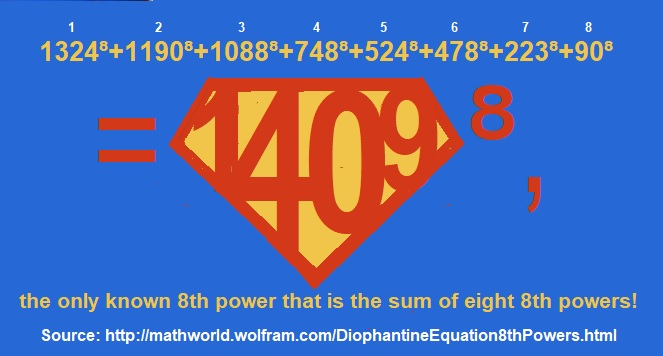Sometimes 1409 wears a more modest super cape because 1409² is also the sum of TWO squares:
159² +1400² = 1409²Here are some more super facts about the number 1409:

• 1409 is a prime number.
• Prime factorization: 1409 is prime.
• 1409 has no exponents greater than 1 in its prime factorization, so √1409 cannot be simplified.
• The exponent in the prime factorization is 1. Adding one to that exponent we get (1 + 1) = 2. Therefore 1409 has exactly 2 factors.
• The factors of 1409 are outlined with their factor pair partners in the graphic below.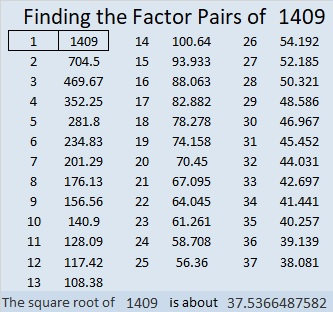How do we know that 1409 is a prime number? If 1409 were not a prime number, then it would be divisible by at least one prime number less than or equal to √1409. Since 1409 cannot be divided evenly by 2, 3, 5, 7, 11, 13, 17, 19, 23, 29 31, or 37, we know that 1409 is a prime number.

1409 is the sum of two squares:
28² + 25² = 1409

1409 is the hypotenuse of a primitive Pythagorean triple:
159-1400-1409 calculated from 28² – 25², 2(28)(25), 28² + 25²

Here’s another way we know that 1409 is a prime number: Since its last two digits divided by 4 leave a remainder of 1, and 28² + 25² = 1409 with 28 and 25 having no common prime factors, 1409 will be prime unless it is divisible by a prime number Pythagorean triple hypotenuse less than or equal to √1409. Since 1409 is not divisible by 5, 13, 17, 29, or 37, we know that 1409 is a prime number.

# 1399 and Level 6

The eligible common factors of 48 and 72 are 6, 8, and 12. The common factors for 10 and 30 are 5 and 10.  Don’t guess and check the possibilities! Can you figure out the logic needed to start this puzzle?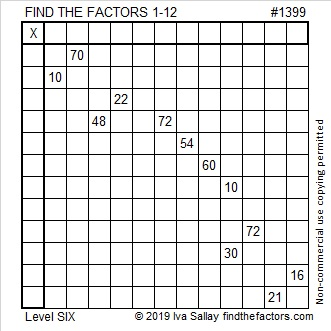Print the puzzles or type the solution in this excel file: 12 Factors 1389-1403

Here’s a little information about the number 1399:

• 1399 is a prime number.
• Prime factorization: 1399 is prime.
• 1399 has no exponents greater than 1 in its prime factorization, so √1399 cannot be simplified.
• The exponent in the prime factorization is 1. Adding one to that exponent we get (1 + 1) = 2. Therefore 1399 has exactly 2 factors.
• The factors of 1399 are outlined with their factor pair partners in the graphic below.How do we know that 1399 is a prime number? If 1399 were not a prime number, then it would be divisible by at least one prime number less than or equal to √1399. Since 1399 cannot be divided evenly by 2, 3, 5, 7, 11, 13, 17, 19, 23, 29 31, or 37, we know that 1399 is a prime number.

1399 is the difference of two squares:
700² – 699² = 1399

# 1381 Hooda Math’s Multiplication Game

The school year is almost over, and class periods were only twenty-five minutes long today. I went online looking for math games that would benefit my students and I found a winner with Hooda Math’s Multiplication Game.If you count the multiplication facts in a 9×9 multiplication table, you will see 99 facts, but many of the products are duplicated in the table. Every yellow square below is also in white elsewhere in the table:There are actually only 36 unique products in the multiplication table above. Hooda Math has cleverly arranged those 36 products in a 6×6 grid that becomes the game board. In this two-person game, students take turns moving one of two arrows to a number from 1 to 9 at the bottom of the screen and claiming the square that contains the product of the numbers. The catch is that players must keep one of the numbers chosen by the previous player and cannot claim a product that has already been claimed by either player. (Player 1 cannot score on his first turn.) One student is green and the other is purple and the first to claim four squares in a row is the winner. The rules on the website are VERY short and simple.

Students played this game today. I played it as well. Sometimes I won, and sometimes I lost, but the losses are more interesting than the wins:

In one game, my opponent took the square that I needed to get four in a row vertically for the win. All she was trying to do was block me from winning, however, when she took that square, the game declared her the winner. We were puzzled why she was the winner until she figured out that making that move gave her four in a row diagonally. That’s when we found out players can win by getting four in a row diagonally as well as vertically or horizontally.

In another game, I had two possible moves that would have made me be the winner. I just needed my opponent to choose a 1, 6, or 8 as their other factor, and I would win with 1 × 1 = 1 or 6 × 8 = 48. Unfortunately, he knew to beware of the numbers that would make me win. One of the arrows was pointing to 5, and he made the other arrow point to 5. By now there were no other products left on the board that were divisible by 5, so I couldn’t win because I couldn’t move either of the arrows.

That’s how I didn’t win the game either of those times, but I had a lot of fun anyway, and you will, too!

This is my 1381st post. Here’s some information about that number:

• 1381 is a prime number.
• Prime factorization: 1381 is prime.
• 1381 has no exponents greater than 1 in its prime factorization, so √1381 cannot be simplified.
• The exponent in the prime factorization is 1. Adding one to that exponent we get (1 + 1) = 2. Therefore 1381 has exactly 2 factors.
• The factors of 1381 are outlined with their factor pair partners in the graphic below.How do we know that 1381 is a prime number? If 1381 were not a prime number, then it would be divisible by at least one prime number less than or equal to √1381. Since 1381 cannot be divided evenly by 2, 3, 5, 7, 11, 13, 17, 19, 23, 29 31, or 37, we know that 1381 is a prime number.

1381 is the 24th Centered Pentagonal Number because
5(23)(24)/2 + 1 = 1381. In formula means that it is one more than five times the 23rd triangular number, as I’ve illustrated below:1381 is the sum of two squares:
34² +15² = 1381

1381 is the hypotenuse of a Pythagorean triple:
931-1020-1381 calculated from 34² -15², 2(34)(15), 34² +15²

Here’s another way we know that 1381 is a prime number: Since its last two digits divided by 4 leave a remainder of 1, and 34² + 15² = 1381 with 34 and 15 having no common prime factors, 1381 will be prime unless it is divisible by a prime number Pythagorean triple hypotenuse less than or equal to √1381. Since 1381 is not divisible by 5, 13, 17,  29, or 37, we know that 1381 is a prime number.

# 1373 and Level 1

This puzzle is really just a multiplication table but the factors are missing and the products are not in order. You can figure out where the factors go, and then the clues will all make sense.Print the puzzles or type the solution in this excel file: 10 Factors 1373-1388

Now I’ll share some facts about the puzzle number, 1373:

• 1373 is a prime number.
• Prime factorization: 1373 is prime.
• 1373 has no exponents greater than 1 in its prime factorization, so √1373 cannot be simplified.
• The exponent in the prime factorization is 1. Adding one to that exponent we get (1 + 1) = 2. Therefore 1373 has exactly 2 factors.
• The factors of 1373 are outlined with their factor pair partners in the graphic below.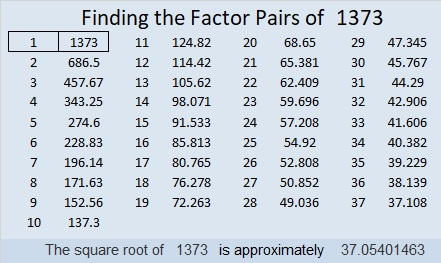How do we know that 1373 is a prime number? If 1373 were not a prime number, then it would be divisible by at least one prime number less than or equal to √1373. Since 1373 cannot be divided evenly by 2, 3, 5, 7, 11, 13, 17, 19, 23, 29 31, or 37, we know that 1373 is a prime number.

1373 is the sum of two squares:
37² + 2² = 1373

1373 is the hypotenuse of a Pythagorean triple:
148-1365-1373 calculated from 2(37)(2), 37² – 2², 37² + 2²

Here’s another way we know that 1373 is a prime number: Since its last two digits divided by 4 leave a remainder of 1, and 37² + 2² = 1373 with 37 and 2 having no common prime factors, 1373 will be prime unless it is divisible by a prime number Pythagorean triple hypotenuse less than or equal to √1373. Since 1373 is not divisible by 5, 13, 17,  29, or 37, we know that 1373 is a prime number.

# 1361 and Level 5

If you carefully use logic instead of guessing and checking you can find the unique solution to this puzzle without tearing your hair out!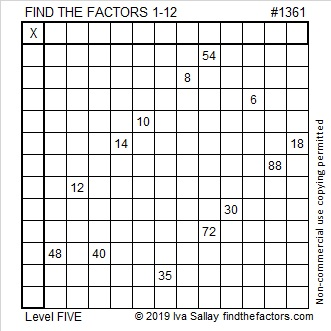Print the puzzles or type the solution in this excel file: 12 Factors 1357-1365

Here are some facts about the number 1361:

• 1361 is a prime number.
• Prime factorization: 1361 is prime.
• 1361 has no exponents greater than 1 in its prime factorization, so √1361 cannot be simplified.
• The exponent in the prime factorization is 1. Adding one to that exponent we get (1 + 1) = 2. Therefore 1361 has exactly 2 factors.
• The factors of 1361 are outlined with their factor pair partners in the graphic below.

How do we know that 1361 is a prime number? If 1361 were not a prime number, then it would be divisible by at least one prime number less than or equal to √1361. Since 1361 cannot be divided evenly by 2, 3, 5, 7, 11, 13, 17, 19, 23, 29 or 31, we know that 1361 is a prime number.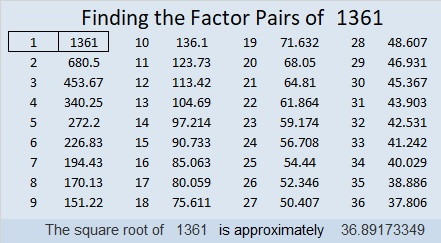1361 is the first prime number after 1327. That was 34 numbers ago!

1361 is the sum of two squares:
31² + 20² = 1361

1361 is the hypotenuse of a Pythagorean triple:
561-1240-1361 calculated from 31² – 20², 2(31)(20), 31² + 20²

Here’s another way we know that 1361 is a prime number: Since its last two digits divided by 4 leave a remainder of 1, and 31² + 20² = 1361 with 31 and 20 having no common prime factors, 1361 will be prime unless it is divisible by a prime number Pythagorean triple hypotenuse less than or equal to √1361. Since 1361 is not divisible by 5, 13, 17, or 29, we know that 1361 is a prime number.

# 1307 and Level 5

This puzzle shows 10 of the 100 products in a 10 × 10 multiplication table. Can you figure out where to put the factors? There’s only one way that works!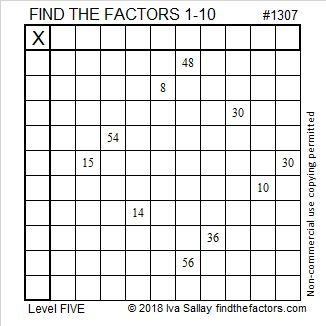Print the puzzles or type the solution in this excel file: 10-factors-1302-1310

Here are some facts about the number 1307:

• 1307 is a prime number.
• Prime factorization: 1307 is prime.
• The exponent of prime number 1307 is 1. Adding 1 to that exponent we get (1 + 1) = 2. Therefore 1307 has exactly 2 factors.
• Factors of 1307: 1, 1307
• Factor pairs: 1307 = 1 × 1307
• 1307 has no square factors that allow its square root to be simplified. √1307 ≈ 36.15245

How do we know that 1307 is a prime number? If 1307 were not a prime number, then it would be divisible by at least one prime number less than or equal to √1307 ≈ 36.2. Since 1307 cannot be divided evenly by 2, 3, 5, 7, 11, 13, 17, 19, 23, 29 or 31, we know that 1307 is a prime number.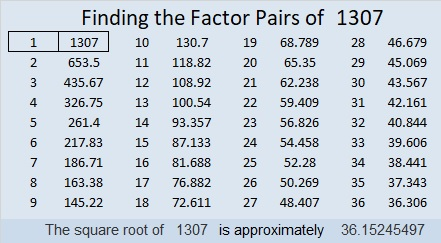1307 is palindrome 797 in BASE 13 because 7(13²) + 9(13) + 7(1) = 1307

# 1303 and Level 2

Multiplication tables usually have facts up to 10 × 10 = 100 or possibly 12 × 12 = 144. Numbers like 64 and 25 appear only once in those multiplication tables. Those two clues can help you get a good start solving this level 2 puzzle.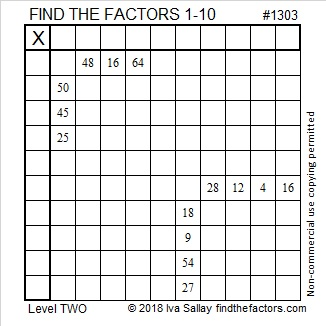Print the puzzles or type the solution in this excel file: 10-factors-1302-1310

Now I’ll share a few facts about the number 1303:

• 1303 is a prime number.
• Prime factorization: 1303 is prime.
• The exponent of prime number 1303 is 1. Adding 1 to that exponent we get (1 + 1) = 2. Therefore 1303 has exactly 2 factors.
• Factors of 1303: 1, 1303
• Factor pairs: 1303 = 1 × 1303
• 1303 has no square factors that allow its square root to be simplified. √1303 ≈ 36.09709

How do we know that 1303 is a prime number? If 1303 were not a prime number, then it would be divisible by at least one prime number less than or equal to √1303 ≈ 36.1. Since 1303 cannot be divided evenly by 2, 3, 5, 7, 11, 13, 17, 19, 23, 29 or 31, we know that 1303 is a prime number.1303 is the sum of three consecutive primes:
431 + 433 + 439 = 1303